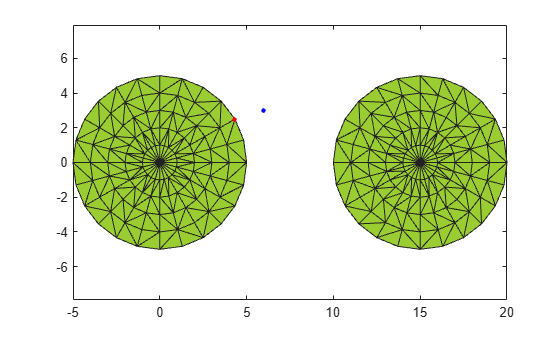# nearestNeighbor

Determine nearest alpha shape boundary point

## Syntax

``I = nearestNeighbor(shp,qx,qy)``
``I = nearestNeighbor(shp,qx,qy,qz)``
``I = nearestNeighbor(shp,QP)``
``I = nearestNeighbor(___,RegionID)``
``````[I,D] = nearestNeighbor(___)``````

## Description

````I = nearestNeighbor(shp,qx,qy)`, for a 2-D alpha shape `shp`, returns the indices of points on the boundary of `shp` closest to the query points. `I` is the array of nearest neighbor indices where each index corresponds to the row index in `shp.Points`. The `qx` and `qy` query coordinates must be the same size.```
````I = nearestNeighbor(shp,qx,qy,qz)`, for a 3-D alpha shape, returns the indices of the boundary points of `shp` closest to `(qx,qy,qz)` and corresponds to the row indices in `shp.Points`. The `qx`, `qy`, and `qz` query coordinates must be the same size.```

example

````I = nearestNeighbor(shp,QP)` specifies the query points as a matrix `QP`. For a 2-D alpha shape, `QP` is a matrix with two columns representing the `qx` and `qy` coordinates. For a 3-D alpha shape, `QP` has three columns representing the `qx`, `qy`, and `qz` coordinates.```
````I = nearestNeighbor(___,RegionID)` returns the index of the nearest point that lies on the boundary of the region specified by `RegionID`, where `1` ≤ `RegionID` ≤ `numRegions(shp)`. You can include any of the input arguments in the previous syntaxes.```
``````[I,D] = nearestNeighbor(___)``` additionally returns the Euclidean distance between the query point and its nearest neighbor. `D` has the same size as `I`.```

## Examples

collapse all

Create a set of 2-D points.

```th = (pi/12:pi/12:2*pi)'; x1 = [reshape(cos(th)*(1:5), numel(cos(th)*(1:5)),1); 0]; y1 = [reshape(sin(th)*(1:5), numel(sin(th)*(1:5)),1); 0]; x = [x1; x1+15]; y = [y1; y1];```

Create and plot an alpha shape with alpha radius equal to 1.

```shp = alphaShape(x,y,1); plot(shp) hold on```Compute the nearest `shp` boundary point to the query point `QP`. Plot the query point in blue and the nearest boundary neighbor in red.

```QP = [6 3]; plot(QP(1),QP(2),'b.','MarkerSize',10) hold on I = nearestNeighbor(shp, QP); plot(shp.Points(I,1),shp.Points(I,2),'r.','MarkerSize',10)```## Input Arguments

collapse all

Alpha shape, specified as an `alphaShape` object. For more information, see `alphaShape`.

Example: `shp = alphaShape(x,y)` creates a 2-D `alphaShape` object from the `(x,y)` point coordinates.

Query point x-coordinates, specified as a numeric array.

Data Types: `double`

Query point y-coordinates, specified as a numeric array.

Data Types: `double`

Query point z-coordinates, specified as a numeric array.

Data Types: `double`

Query point coordinates, specified as a two-column matrix or a three-column matrix.

• For 2-D, the columns of `P` represent `qx` and `qy` coordinates, respectively.

• For 3-D, the columns of `P` represent `qx`, `qy`, and `qz` coordinates, respectively.

Data Types: `double`

ID number for region in alpha shape, specified as a positive integer scalar between `1` and `numRegions(shp)`.

An alpha shape can contain several smaller regions, depending on the point set and parameters. Each of these smaller regions is assigned a unique `RegionID`, which numbers the regions from the largest area or volume to the smallest. For example, consider a 3-D alpha shape with two regions. The region with the largest volume has a `RegionID` of 1, and the smaller region has a `RegionID` of 2.

Example: `shp.RegionThreshold = area(shp,numRegions(shp)-2);` suppresses the two smallest regions in 2-D alpha shape `shp`.

Data Types: `double`

## Output Arguments

collapse all

Nearest neighbor indices, returned as an integer-valued array. The indices correspond to the row index of `shp.Points` and indicate the points on the boundary of `shp` that are closest to the given query points.

Distance from query points to nearest neighbors, returned as a numeric array. `D` is the 2-D or 3-D Euclidean distance and is the same size as `I`.

## Version History

Introduced in R2015a